# 5 Model comparisons and testing for lack of fit

## 5.1 F-tests for comparing two models

### 5.1.1 Example:

Model A: $$\mathbb{E}[y] = \beta_0 + \beta_1 x_1$$

Model B: $$\mathbb{E}[y] = \beta_0 + \beta_1 x_1 + \beta_2 x_2 + \beta_3 x_3$$

i.e. in Model A, $$\beta_2=\beta_3=0$$.

Model A is the reduced or simpler model and model B is the full model.

The $$\mbox{SSE}$$ for Model B will be smaller than the $$\mbox{SSE}$$ for Model A but is the reduction enough to justify the two extra parameters?

We have:

Model A:

$\mbox{SST} = \mbox{SSR}(A) + \mbox{SSE}(A)$

Model B:

$\mbox{SST} = \mbox{SSR}(B) + \mbox{SSE}(B)$

Note:

$\mbox{SSE}(A)-\mbox{SSE}(B)=\mbox{SSR}(B)-\mbox{SSR}(A)$

### 5.1.2 F-test to compare models:

Model A: $$\mathbb{E}[y] = \beta_0 + \beta_1 x_1 + ... + \beta_q x_q$$

Model B: $$\mathbb{E}[y] = \beta_0 + \beta_1 x_1 + ... + \beta_k x_k$$

where $$q<k$$ and Model A is nested within Model B.

$$H_0$$: $$\beta_{q+1} = \beta_{q+2} = ... = \beta_k = 0$$

$$H_A$$: At least one $$\beta_{q+1}, ... , \beta_k \neq 0.$$

$F =\frac{(\mbox{SSE}(A)-\mbox{SSE}(B))/(k-q)}{\mbox{SSE}(B)/(n-p)}.$

Under $$H_0$$, $F \sim F_{(k-q),(n-p)},$ where $$p = (k+1).$$

Note: Equivalently, the F-test can be written as:

$F =\frac{(\mbox{SSR}(B)-\mbox{SSR}(A))/(k-q)}{\mbox{SSE}(B)/(n-p)}.$

Note: Models A and B must be hierarchical for the F-test to be valid.

### 5.1.3 Example: Steam data

This data is from a study undertaken to understand the factors that caused energy consumption in detergent manufacturing over a 25 month period. Example from Draper and Smith (1966).

The data variables are:

y = STEAM Pounds of steam used monthly.

x1 = TEMP Average atmospheric temperature ($$^o$$F).

x2 = INV Inventory: pounds of real fatty acid in storage per month.

x3 = PROD Pounds of crude glycerin made.

x4 = WIND Average wind velocity (in mph).

x5 = CDAY Calendar days per month.

x6 = OPDAY Operating days per month.

x7 = FDAY Days below $$32^o$$F.

x8 = WIND2 Average wind velocity squared.

x9 = STARTS Number of production start-ups during the month.

## The following objects are masked from steamdata (pos = 9):
##
##     CDAY, FDAY, INV, OPDAY, PROD, STARTS, STEAM, TEMP, WIND, WIND2
## The following objects are masked from steamdata (pos = 16):
##
##     CDAY, FDAY, INV, OPDAY, PROD, STARTS, STEAM, TEMP, WIND, WIND2
## The following objects are masked from steamdata (pos = 23):
##
##     CDAY, FDAY, INV, OPDAY, PROD, STARTS, STEAM, TEMP, WIND, WIND2
## The following objects are masked from steamdata (pos = 30):
##
##     CDAY, FDAY, INV, OPDAY, PROD, STARTS, STEAM, TEMP, WIND, WIND2
## The following objects are masked from steamdata (pos = 37):
##
##     CDAY, FDAY, INV, OPDAY, PROD, STARTS, STEAM, TEMP, WIND, WIND2
## The following objects are masked from steamdata (pos = 44):
##
##     CDAY, FDAY, INV, OPDAY, PROD, STARTS, STEAM, TEMP, WIND, WIND2
## The following objects are masked from steamdata (pos = 51):
##
##     CDAY, FDAY, INV, OPDAY, PROD, STARTS, STEAM, TEMP, WIND, WIND2
## The following objects are masked from steamdata (pos = 59):
##
##     CDAY, FDAY, INV, OPDAY, PROD, STARTS, STEAM, TEMP, WIND, WIND2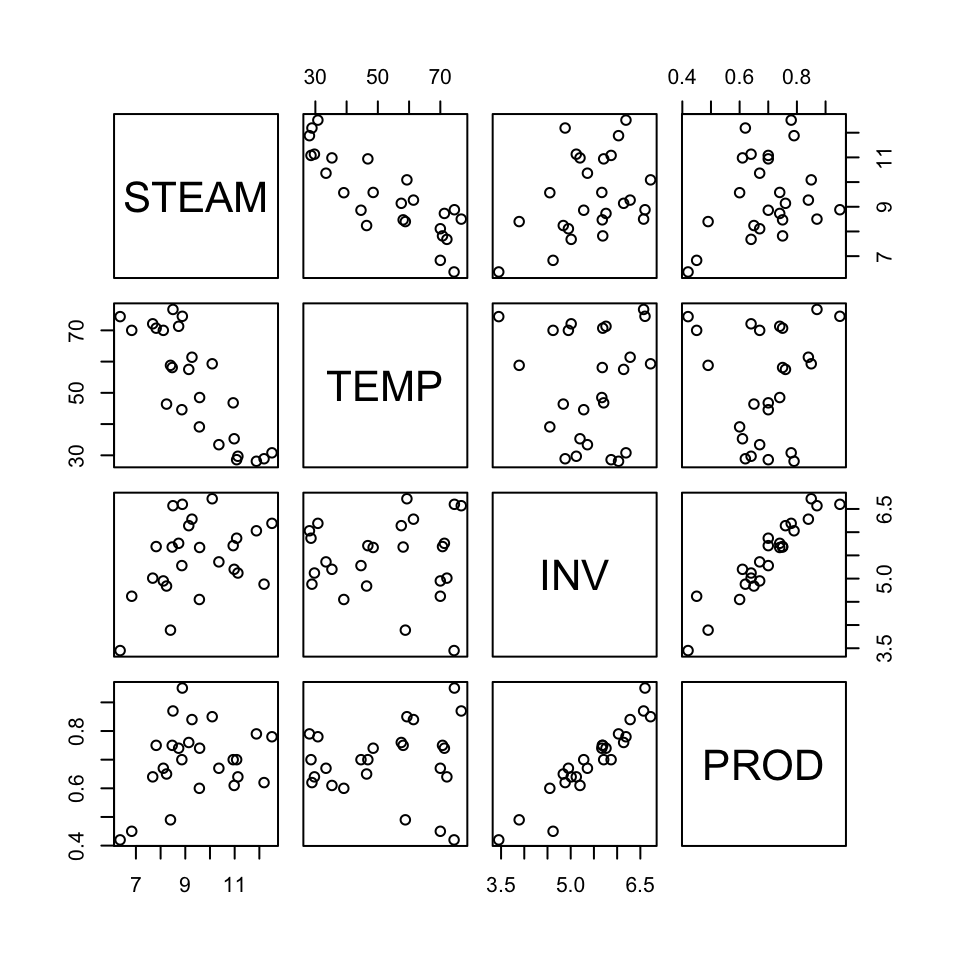Model A: $$\mathbb{E}[y] = \beta_0 + \beta_1 x_1$$

Model B: $$\mathbb{E}[y] = \beta_0 + \beta_1 x_1 + \beta_2 x_2 + \beta_3 x_3$$

where $$x_1$$ = TEMP, $$x_2$$ = INV, $$x_3$$ = PROD.

modelA <- lm(STEAM ~ TEMP)
modelB <- lm(STEAM ~ TEMP + INV + PROD)
summary(modelA)
##
## Call:
## lm(formula = STEAM ~ TEMP)
##
## Residuals:
##     Min      1Q  Median      3Q     Max
## -1.6789 -0.5291 -0.1221  0.7988  1.3457
##
## Coefficients:
##             Estimate Std. Error t value Pr(>|t|)
## (Intercept) 13.62299    0.58146  23.429  < 2e-16 ***
## TEMP        -0.07983    0.01052  -7.586 1.05e-07 ***
## ---
## Signif. codes:  0 '***' 0.001 '**' 0.01 '*' 0.05 '.' 0.1 ' ' 1
##
## Residual standard error: 0.8901 on 23 degrees of freedom
## Multiple R-squared:  0.7144, Adjusted R-squared:  0.702
## F-statistic: 57.54 on 1 and 23 DF,  p-value: 1.055e-07
summary(modelB)
##
## Call:
## lm(formula = STEAM ~ TEMP + INV + PROD)
##
## Residuals:
##     Min      1Q  Median      3Q     Max
## -1.2348 -0.4116  0.1240  0.3744  1.2979
##
## Coefficients:
##              Estimate Std. Error t value Pr(>|t|)
## (Intercept)  9.514814   1.062969   8.951 1.30e-08 ***
## TEMP        -0.079928   0.007884 -10.138 1.52e-09 ***
## INV          0.713592   0.502297   1.421     0.17
## PROD         0.330497   3.267694   0.101     0.92
## ---
## Signif. codes:  0 '***' 0.001 '**' 0.01 '*' 0.05 '.' 0.1 ' ' 1
##
## Residual standard error: 0.652 on 21 degrees of freedom
## Multiple R-squared:  0.8601, Adjusted R-squared:  0.8401
## F-statistic: 43.04 on 3 and 21 DF,  p-value: 3.794e-09
anova(modelA)
## Analysis of Variance Table
##
## Response: STEAM
##           Df Sum Sq Mean Sq F value    Pr(>F)
## TEMP       1 45.592  45.592  57.543 1.055e-07 ***
## Residuals 23 18.223   0.792
## ---
## Signif. codes:  0 '***' 0.001 '**' 0.01 '*' 0.05 '.' 0.1 ' ' 1
anova(modelB)
## Analysis of Variance Table
##
## Response: STEAM
##           Df Sum Sq Mean Sq  F value    Pr(>F)
## TEMP       1 45.592  45.592 107.2523 1.046e-09 ***
## INV        1  9.292   9.292  21.8588 0.0001294 ***
## PROD       1  0.004   0.004   0.0102 0.9203982
## Residuals 21  8.927   0.425
## ---
## Signif. codes:  0 '***' 0.001 '**' 0.01 '*' 0.05 '.' 0.1 ' ' 1

$$H_0$$: $$\beta_2 = \beta_3 = 0$$

$$H_A$$: At least one $$\beta_2, \beta_3 \neq 0$$

\begin{align*} F_{obs} & = \frac{(\mbox{SSE}(A)-\mbox{SSE}(B))/(k-q)}{\mbox{SSE}(B)/(n-p)}\\ & = \frac{(18.223-8.927)/(3-1)}{8.927/(25-4)}=10.93.\\ \end{align*}

$$F_{(0.05,2,21)} = 3.467$$, $$F_{(0.01,2,21)} = 5.780$$

P-value $$<0.01$$, we reject $$H_0$$ and conclude that at least one of $$\beta_2$$, $$\beta_3$$ differ from 0.

anova(modelA, modelB)
## Analysis of Variance Table
##
## Model 1: STEAM ~ TEMP
## Model 2: STEAM ~ TEMP + INV + PROD
##   Res.Df    RSS Df Sum of Sq      F    Pr(>F)
## 1     23 18.223
## 2     21  8.927  2    9.2964 10.934 0.0005569 ***
## ---
## Signif. codes:  0 '***' 0.001 '**' 0.01 '*' 0.05 '.' 0.1 ' ' 1

## 5.2 Sequential sums of squares

### 5.2.1 Example:

Model A: $$\mathbb{E}[y] = \beta_0 + \beta_1 x_1$$

Model B: $$\mathbb{E}[y] = \beta_0 + \beta_1 x_1 + \beta_2 x_2+ \beta_3 x_3$$

As noted earlier, the reduction in $$\mbox{SSE}$$ going from Model A to B, is equivalent to the increase in $$\mbox{SSR}$$, i.e. $\mbox{SSE}(A)-\mbox{SSE}(B)=\mbox{SSR}(B)-\mbox{SSR}(A).$

We can denote: $\mbox{SSR}(B|A)=\mbox{SSR}(B)-\mbox{SSR}(A).$

These are the sequential sums of squares. We can write: \begin{align*} \mbox{SST} & = \mbox{SSR}(B) + \mbox{SSE}(B)\\ & = \mbox{SSR}(A) +\mbox{SSR}(B) - \mbox{SSR}(A) + \mbox{SSE}(B)\\ & = \mbox{SSR}(A) + \mbox{SSR}(B|A) + \mbox{SSE}(B).\\ \mbox{SST} - \mbox{SSE}(B) &= \mbox{SSR}(A) + \mbox{SSR}(B|A)\\ \mbox{SSR}(B) &= \mbox{SSR}(A) + \mbox{SSR}(B|A).\\ \end{align*}

If model A is appropriate, $$\mbox{SSR}(B|A)$$ should be small.

### 5.2.2 Example: Steam data

Model A: $$\mathbb{E}[y] = \beta_0$$

Model B: $$\mathbb{E}[y] = \beta_0 + \beta_1 x_1$$

Model C: $$\mathbb{E}[y] = \beta_0 + \beta_1 x_1 + \beta_2 x_2$$

Model D: $$\mathbb{E}[y] = \beta_0 + \beta_1 x_1 + \beta_2 x_2 + \beta_3 x_3$$

SOURCE df seqSS Notation
TEMP 1 45.592 SSR(B|A)
INV 1 9.292 SSR(C|B)
PROD 1 0.004 SSR(D|C)

From the ANOVA table,

\begin{align*} \mbox{SSR}(D)& =54.889\\ & = \mbox{SSR}(B|A) + \mbox{SSR}(C|B) + \mbox{SSR}(D|C)\\ \end{align*}

We can use the F-test for comparing two models to test Seq SS.

1):

Model A: $$\mathbb{E}[y] = \beta_0$$

Model D: $$\mathbb{E}[y] = \beta_0 + \beta_1 x_1 + \beta_2 x_2 + \beta_3 x_3$$

$$H_0$$: $$\beta_1 = \beta_2 = \beta_3 = 0$$

$$H_a$$: Not all $$\beta_i$$ are 0

$$\mbox{SSR}(A) = 0$$

$$\mbox{SSR}(D|A) = \mbox{SSR}(D) = 54.889.$$

$F_{obs} = \frac{\mbox{SSR}(D|A)/(k-q)}{\mbox{SSE}(D)/(n-p)} = \frac{54.889/(3-0)}{8.927/(25-4)}=43.04$

P-value $$< 0.001$$, we reject $$H_0$$ and conclude that not all $$\beta_i$$ are 0.

2):

Model C: $$\mathbb{E}[y] = \beta_0 + \beta_1 x_1 + \beta_2 x_2$$

Model D: $$\mathbb{E}[y] = \beta_0 + \beta_1 x_1 + \beta_2 x_2 + \beta_3 x_3$$

$$H_0$$: $$\beta_3 = 0$$

$$H_a$$: $$\beta_3 \neq 0$$

$$\mbox{SSR}(D|C) = 0.004$$

$F_{obs} = \frac{\mbox{SSR}(D|C)/(k-q)}{\mbox{SSE}(D)/(n-p)} = \frac{0.004/1}{8.927/21} = 0.01$

$$F_{(0.1,1,21)} = 2.96096$$, so P-value $$>0.05$$.

We fail to reject $$H_0$$ and conclude there is no evidence $$\beta_3 \neq 0$$, i.e. $$x_3$$ is not needed in the model.

This F-test is equivalent to a t-test for $$\beta_3$$: $T = 0.1$ $F = (0.1)(0.1) = 0.01$

The p-value for both tests $$= 0.92$$.

Note: The Seq SS values depend on the order in which the variables are added to the model (unless the variables are uncorrelated).

### 5.2.3 Example: Steam cont’d in MTB

Regression Analysis: STEAM versus TEMP

Analysis of Variance

Regression      1  45.5924  45.5924    57.54    0.000
TEMP            1  45.5924  45.5924    57.54    0.000
Error          23  18.2234   0.7923
Lack-of-Fit    22  17.4042   0.7911     0.97    0.680
Pure Error      1   0.8192   0.8192
Total          24  63.8158

Model Summary

0.890125  71.44%     70.20%      66.32%

Coefficients

Term         Coef  SE Coef  T-Value  P-Value   VIF
Constant   13.623    0.581    23.43    0.000
TEMP      -0.0798   0.0105    -7.59    0.000  1.00

Regression Equation

STEAM = 13.623 -0.0798TEMP
  Regression Analysis: STEAM versus TEMP, INV, PROD

Analysis of Variance

Regression   3  54.8888  18.2963    43.04    0.000
TEMP         1  43.6895  43.6895   102.78    0.000
INV          1   0.8580   0.8580     2.02    0.170
PROD         1   0.0043   0.0043     0.01    0.920
Error       21   8.9270   0.4251
Total       24  63.8158

Model Summary

0.651993  86.01%     84.01%      79.77%

Coefficients

Term          Coef  SE Coef  T-Value  P-Value   VIF
Constant      9.51     1.06     8.95    0.000
TEMP      -0.07993  0.00788   -10.14    0.000  1.05
INV          0.714    0.502     1.42    0.170  9.51
PROD          0.33     3.27     0.10    0.920  9.55

Regression Equation

STEAM = 9.51 -0.07993TEMP +0.714INV +0.33PROD



Regression $$>$$ Options $$>$$ sum of squares tests $$>$$ sequential


Regression Analysis: STEAM versus TEMP, INV, PROD

Analysis of Variance

Source      DF   Seq SS   Seq MS  F-Value  P-Value
Regression   3  54.8888  18.2963    43.04    0.000
TEMP         1  45.5924  45.5924   107.25    0.000
INV          1   9.2921   9.2921    21.86    0.000
PROD         1   0.0043   0.0043     0.01    0.920
Error       21   8.9270   0.4251
Total       24  63.8158

Model Summary

0.651993  86.01%     84.01%      79.77%

Coefficients

Term          Coef  SE Coef  T-Value  P-Value   VIF
Constant      9.51     1.06     8.95    0.000
TEMP      -0.07993  0.00788   -10.14    0.000  1.05
INV          0.714    0.502     1.42    0.170  9.51
PROD          0.33     3.27     0.10    0.920  9.55

Regression Equation

STEAM = 9.51 - 0.07993TEMP + 0.714INV + 0.33PROD

Regression $$>$$ Options $$>$$ sum of squares tests $$>$$ sequential, but change the order in which the predictors are input in MTB. Output below is MTB17, MTB18 rearranges them in the order TEMP INV PROD.

  Regression Analysis: STEAM versus PROD, INV, TEMP

Analysis of Variance

Source      DF  Seq SS   Seq MS  F-Value  P-Value
Regression   3  54.889  18.2963    43.04    0.000
PROD         1   5.958   5.9577    14.02    0.001
INV          1   5.242   5.2415    12.33    0.002
TEMP         1  43.690  43.6895   102.78    0.000
Error       21   8.927   0.4251
Total       24  63.816

Model Summary

0.651993  86.01%     84.01%      79.77%

Coefficients

Term          Coef  SE Coef  T-Value  P-Value   VIF
Constant      9.51     1.06     8.95    0.000
PROD          0.33     3.27     0.10    0.920  9.55
INV          0.714    0.502     1.42    0.170  9.51
TEMP      -0.07993  0.00788   -10.14    0.000  1.05

Regression Equation

STEAM = 9.51 + 0.33PROD + 0.714INV - 0.07993TEMP

The anova and aov functions in R implement a sequential sum of squares (type I). Function Anova(, type= 2) in library(car) gives the adjusted SS (type II)

modelB <- lm(STEAM ~ TEMP + INV + PROD)

anova(modelB)
## Analysis of Variance Table
##
## Response: STEAM
##           Df Sum Sq Mean Sq  F value    Pr(>F)
## TEMP       1 45.592  45.592 107.2523 1.046e-09 ***
## INV        1  9.292   9.292  21.8588 0.0001294 ***
## PROD       1  0.004   0.004   0.0102 0.9203982
## Residuals 21  8.927   0.425
## ---
## Signif. codes:  0 '***' 0.001 '**' 0.01 '*' 0.05 '.' 0.1 ' ' 1
anova(lm(STEAM ~ PROD  + INV + TEMP))
## Analysis of Variance Table
##
## Response: STEAM
##           Df Sum Sq Mean Sq F value    Pr(>F)
## PROD       1  5.958   5.958  14.015  0.001197 **
## INV        1  5.242   5.242  12.330  0.002076 **
## TEMP       1 43.690  43.690 102.776 1.524e-09 ***
## Residuals 21  8.927   0.425
## ---
## Signif. codes:  0 '***' 0.001 '**' 0.01 '*' 0.05 '.' 0.1 ' ' 1
#library(car)
Anova(modelB, type= 2)
## Anova Table (Type II tests)
##
## Response: STEAM
##           Sum Sq Df  F value    Pr(>F)
## TEMP      43.690  1 102.7760 1.524e-09 ***
## INV        0.858  1   2.0183    0.1701
## PROD       0.004  1   0.0102    0.9204
## Residuals  8.927 21
## ---
## Signif. codes:  0 '***' 0.001 '**' 0.01 '*' 0.05 '.' 0.1 ' ' 1

## 5.3 Testing for lack of fit

When replicate values of response are available at some or all of the $$X$$ values, a formal test of model adequacy is available.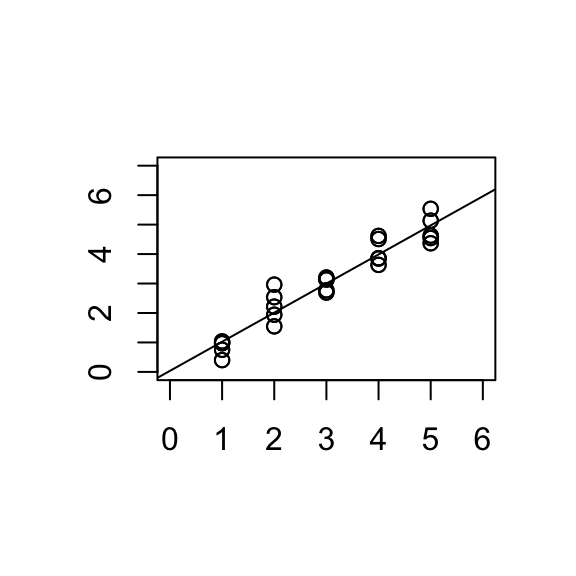The test is based on comparing the fitted value to the average response for that level of $$X$$.

NOTATION: Suppose there are $$g$$ different values of $$X$$ and at the $$i^{th}$$ of these, there are $$n_i$$ observations of $$Y$$.

Let $$\bar{y}_{i.}=\frac{1}{n_i}\sum_{j=1}^{n_i} y_{ij}$$, $$\quad i=1, ..., g.$$

Note: this is the estimate of the group means in the 1-way ANOVA model (means model): $$y_{ij} = \mu_{i} + \epsilon_{ij}$$, where $$\epsilon_{ij}$$ iid $$N(0, \sigma^2)$$.

Then the pure error sums of squares,

\begin{align*} \mbox{SS}_{\mbox{PE}}& =\sum_{i=1}^g \sum_{j=1}^{n_i} (y_{ij}- \bar{y}_{i.})^2\\ df_{PE} & = \sum_{i=1}^g (n_i-1)=n-g, \hspace{1cm} \mbox{where } n=n_1+...+n_g.\\ \end{align*}

Therefore

$\frac{\sum_{i=1}^g \sum_{j=1}^{n_i} (y_{ij}- \bar{y}_{i.})^2}{n-g}$

is an estimator of $$\sigma^2$$.

NOTE:

• Here we use the replicates to obtain an estimate of $$\sigma^2$$ which is independent of the fitted model (SLR).

*This estimator of $$\sigma^2$$ corresponds to the $$\mbox{MSE}$$ in the ANOVA table for the 1-way ANOVA model.

• The 1-way ANOVA model has $$g$$ parameters. The SLR model has $$2$$ parameters. The latter is more restrictive as it requires linearity.

• $$df_{PE} = n-g$$,

• $$df_{SLR} = n-2$$.

The SLR model has a residual SS which is $$\geq$$ residual SS from the means model, i.e. $$\mbox{SSE} \geq \mbox{SS}_{\mbox{PE}}$$.

A large difference $$\mbox{SSE} - \mbox{SS}_{\mbox{PE}}$$ indicates lack of fit of the regression line.

$$\mbox{SS}(\mbox{lack of fit})= \mbox{SSE} - \mbox{SS}_{\mbox{PE}} = \sum_{i,j} (\hat{y}_{i,j} - \bar{y}_i)^2$$, the sum of squared distances of between the SLR estimate and the means model estimate of $$\mathbb{E}(Y_{i,j})$$.

Lack of fit is tested by the statistic:

$F_{obs}=\frac{\left ( \mbox{SSE}-\mbox{SS}_{\mbox{PE}} \right )/(g-2)}{\mbox{SS}_{\mbox{PE}}/(n-g)}.$

$$H_0$$: Regression model fits well

$$H_A$$: Regression model displays lack of fit

Under $$H_0$$, $$F_{obs} \sim F_{g-2,n-g}$$.

Note: This generalises to multiple predictors - the pure error estimate of $$\sigma^2$$ is based on SS between $$y_i$$ for cases with the same values on all predictors. $$df_{SLR} = p$$ instead of 2.

Reject for large values of $$F_{obs}$$.

### 5.3.1 Example: Voltage

Example from Ramsey and Schafer (2002) (case0802 in library(Sleuth3)).

Batches of electrical fluids were subjected to constant voltages until the insulating properties of the fluid broke down.

$$Y$$: time to breakdown

$$X$$: Voltage

The scatterplot of $$Y$$ vs. $$X$$ shows evidence of non-linearity and non-constant variance. The response was log transformed to resolve this.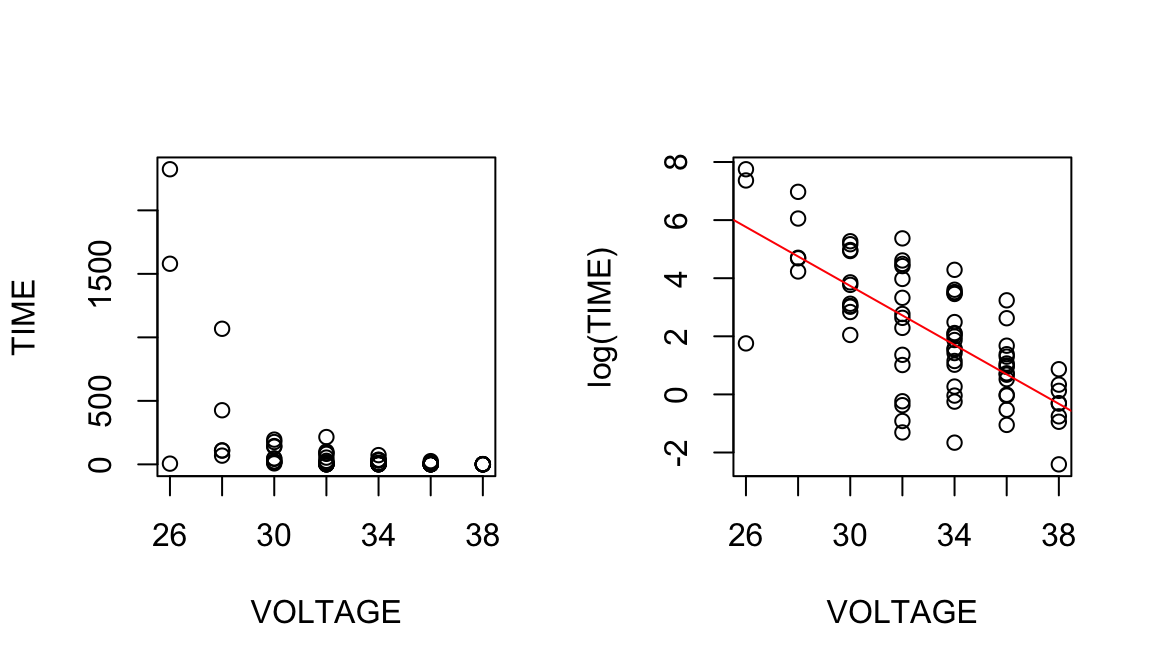$$H_0: \beta_1=0$$

$$H_A: \beta_1 \neq 0$$

$$F = 78.4$$, $$p<0.001$$. We reject $$H_0$$ and conclude that $$\beta_1 \neq 0$$.

$$H_0:$$ S.L.R model is appropriate/correct model

$$H_A:$$ S.L.R model has lack of fit.

$F=\frac{(180.07-173.75)/(7-2)}{173.75/(76-7)}=0.5$

$$F=0.50, p=0.773$$. We conclude that there is no evidence of lack of fit.

One-way ANOVA: LOG_TIME versus CODE

Analysis of Variance

CODE     6   196.5  32.746    13.00    0.000
Error   69   173.7   2.518
Total   75   370.2

Model Summary

1.58685  53.07%     48.99%      38.72%


Regression Analysis: LOG_TIME versus VOLTAGE

Analysis of Variance

Regression      1  190.151  190.151    78.14    0.000
VOLTAGE         1  190.151  190.151    78.14    0.000
Error          74  180.075    2.433
Lack-of-Fit     5    6.326    1.265     0.50    0.773
Pure Error     69  173.749    2.518
Total          75  370.226

Model Summary

1.55995  51.36%     50.70%      48.50%

Regression Equation

LOG_TIME = 18.96 - 0.5074 VOLTAGE

R code

anova(lm(log(TIME)~VOLTAGE))
## Analysis of Variance Table
##
## Response: log(TIME)
##           Df Sum Sq Mean Sq F value   Pr(>F)
## VOLTAGE    1 190.15 190.151  78.141 3.34e-13 ***
## Residuals 74 180.07   2.433
## ---
## Signif. codes:  0 '***' 0.001 '**' 0.01 '*' 0.05 '.' 0.1 ' ' 1
anova(lm(log(TIME)~as.factor(VOLTAGE)))
## Analysis of Variance Table
##
## Response: log(TIME)
##                    Df Sum Sq Mean Sq F value    Pr(>F)
## as.factor(VOLTAGE)  6 196.48  32.746  13.004 8.871e-10 ***
## Residuals          69 173.75   2.518
## ---
## Signif. codes:  0 '***' 0.001 '**' 0.01 '*' 0.05 '.' 0.1 ' ' 1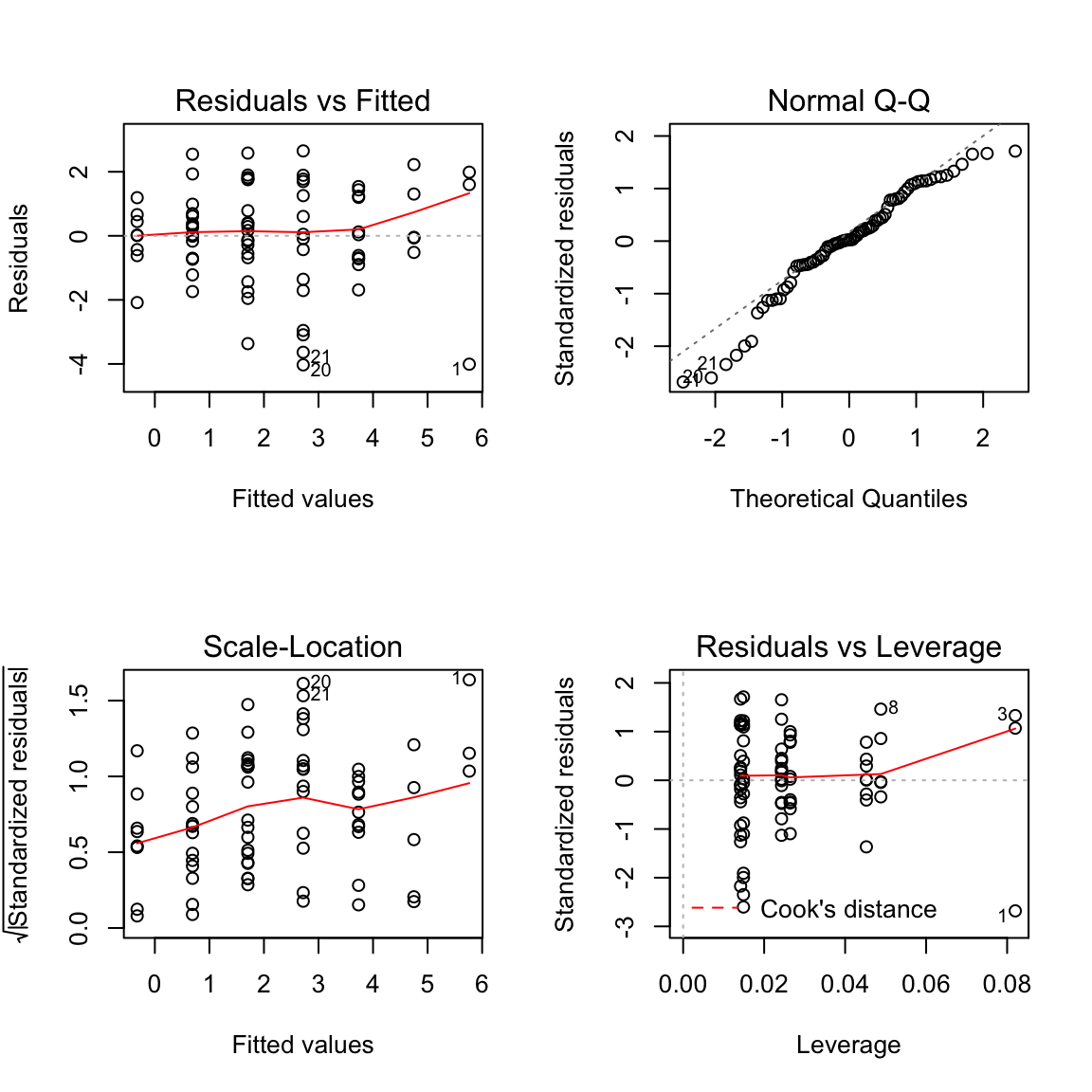In simple linear regression we can assess the importance of a predictor by:

• t-statistic
• $$\mbox{SSR}$$
• $$R^2$$
• $$Y$$-$$X$$ plot.

The analogues in multiple regression for assessing the importance of a predictor in the presence of other predictors are:

• t-statistic
• Seq/Extra SS
• partial $$R^2$$

### 5.4.1 Example: STEAM vs. TEMP, INV, PROD

Model A: $$\mathbb{E}[y] = \beta_0 + \beta_1 x_1+ \beta_2 x_2$$

Model B: $$\mathbb{E}[y] = \beta_0 + \beta_1 x_1 + \beta_2 x_2 + \beta_3 x_3$$

where $$x_1$$ = TEMP, $$x_2$$ = INV, $$x_3$$ = PROD.

• The t-statistic for PROD is small: $$T=0.10, p=0.920$$

• $$\mbox{SSR}(B|A) = 0.004$$ is also small.

• The partial $$R^2$$ for PROD is the proportion of variability in the response unexplained by TEMP and INV that is explained by PROD

\begin{align*} R^2(\mbox{PROD|TEMP, INV})& =\frac{\mbox{SSR}(B|A)}{\mbox{SSE}(A)} & = \frac{0.004}{8.931} = 0.00045=0.045\%\\ \end{align*}
• The added variable plot shows the relationship between a response and a predictor, adjusting for other predictors in the model.

‘Adjusting’ $$Y$$ for predictors $$X_1,...,X_k$$ is achieved by computing the residuals from the regression of $$Y$$ on $$X_1,...,X_k$$. The resulting residuals can be thought of as $$Y$$ with the effect of $$X_1,...,X_k$$ removed.

### 5.4.2 Example: Added variable plot for PROD.

i.e. should we add PROD to the model containing the predictors TEMP and INV? (Response is STEAM).

• Compute $$e$$(STEAM$$|$$ TEMP, INV), i.e. the residuals from regression of STEAM on TEMP and INV.
• Compute $$e$$(PROD$$|$$ TEMP, INV), i.e. the residuals from regression of PROD on TEMP and INV.
• AVP for PROD: Plot $$e$$(STEAM$$|$$ TEMP, INV) vs. $$e$$(PROD$$|$$ TEMP, INV).

We can also do:

AVP INV: Plot $$e$$(STEAM$$|$$ TEMP, PROD) vs. $$e$$(INV$$|$$ TEMP, PROD) AVP TEMP: Plot $$e$$(STEAM$$|$$ INV, PROD) vs. $$e$$(TEMP$$|$$ INV, PROD)

### 5.4.3 Example: Steam data cont’d

fit1 <- lm(STEAM ~ TEMP + INV)
fit2 <- lm(PROD ~ TEMP + INV)
summary(lm(resid(fit1)~ resid(fit2)))
##
## Call:
## lm(formula = resid(fit1) ~ resid(fit2))
##
## Residuals:
##     Min      1Q  Median      3Q     Max
## -1.2348 -0.4116  0.1240  0.3744  1.2979
##
## Coefficients:
##              Estimate Std. Error t value Pr(>|t|)
## (Intercept) 4.487e-17  1.246e-01   0.000    1.000
## resid(fit2) 3.305e-01  3.122e+00   0.106    0.917
##
## Residual standard error: 0.623 on 23 degrees of freedom
## Multiple R-squared:  0.0004869,  Adjusted R-squared:  -0.04297
## F-statistic: 0.0112 on 1 and 23 DF,  p-value: 0.9166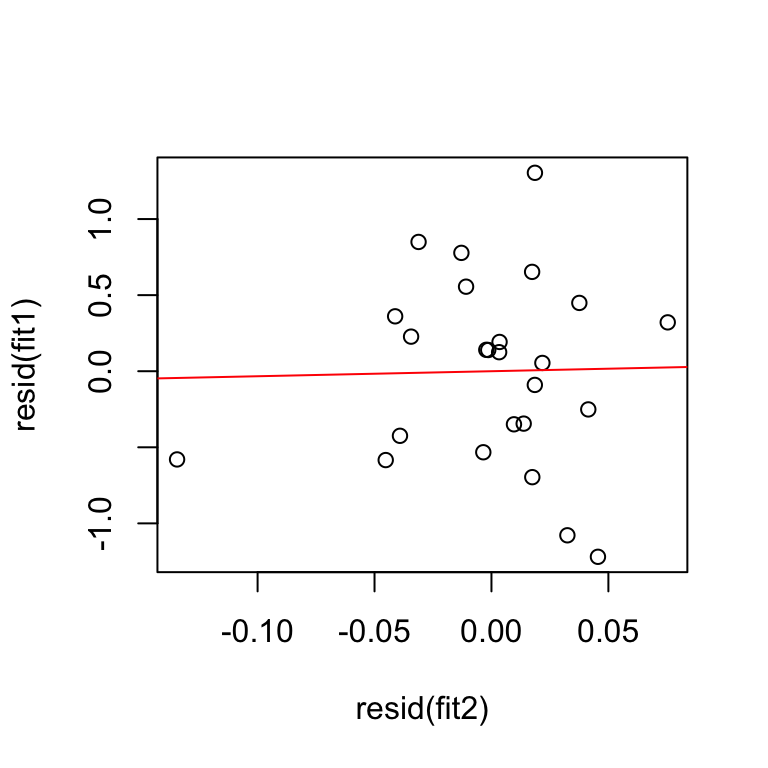Alternatively you can use the avPlots function in the library(car). In minitab use STORAGE option to save the residuals of both models and make a scatterplot.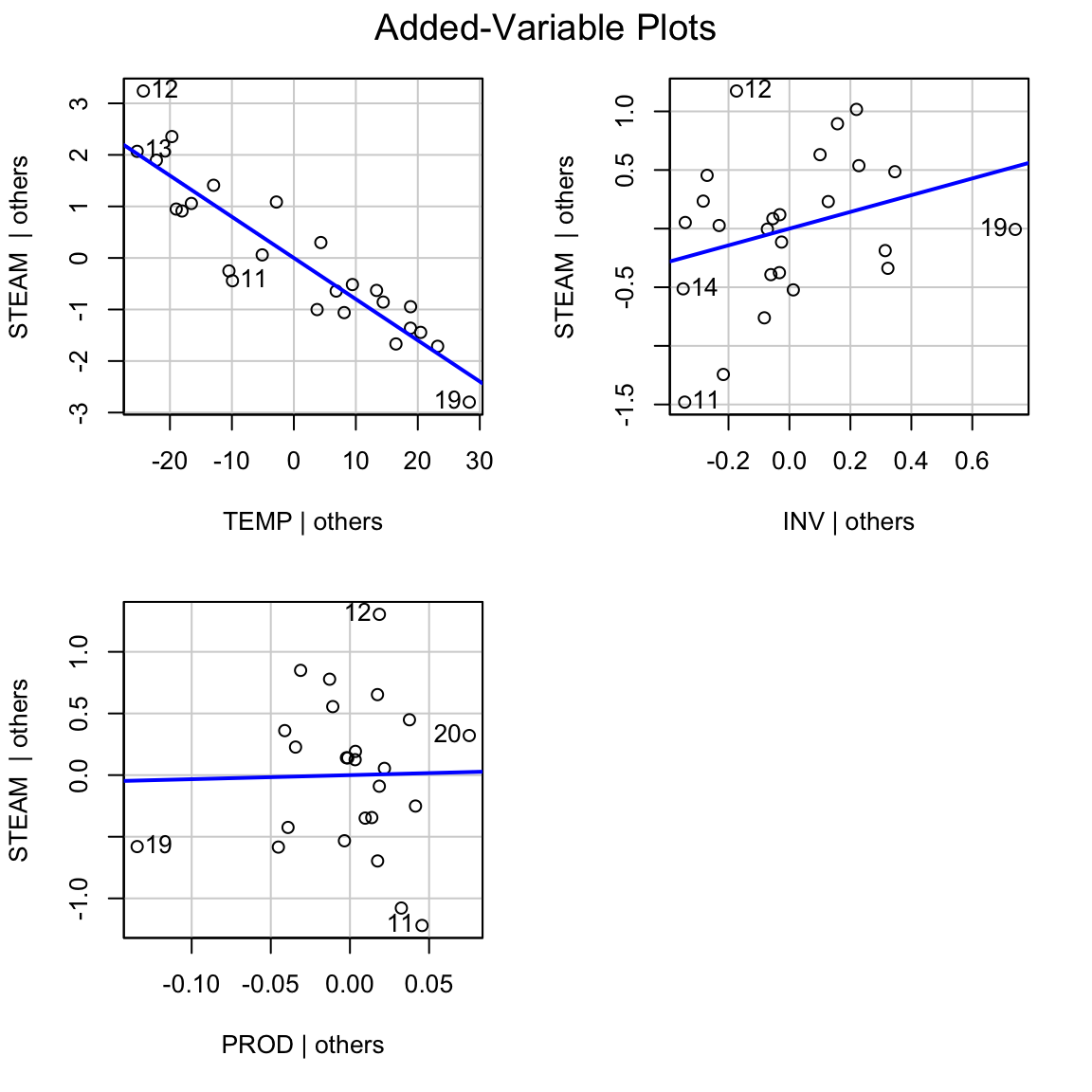### 5.4.4 Properties of AVPs:

• Estimated intercept is 0.

• Slope of the line in AVP for PROD equals $$\hat{\beta}$$ (the coefficient of PROD in the model with TEMP, INV and PROD as predictors.

• Residuals in AVP equal residuals from regression of STEAM on TEMP, INV and PROD.

• $$R^2$$ in AVP for PROD is the partial $$R^2$$ for PROD, i.e. $$R^2$$(PROD$$|$$TEMP,INV).

• $$\hat{\sigma}^2$$ from AVP for PROD $$\approx \hat{\sigma}^2$$ from full model.

$\hat{\sigma}^2_{AVP}(n-2) = \hat{\sigma}^2_{full}(n-p)$

The points in an AVP are clustered tightly around a line if and only if the variable is important.

AV plots may also show outliers, or if the apparent adjusted association between $$Y$$ and $$X_j$$ is due to an influence point.

## 5.5 Visualising Models in Hdim: added variable plots for the bodyfat data.

Bodyfat data from assignment 3: http://rpubs.com/kdomijan/431176

### References

Draper, Norman Richard, and Harry Smith. 1966. Applied Regression Analysis. Wiley Series in Probability and Mathematical Statistics. Wiley.

Ramsey, Fred, and Daniel Schafer. 2002. The Statistical Sleuth: A Course in Methods of Data Analysis. 2nd ed. Duxbury Press.